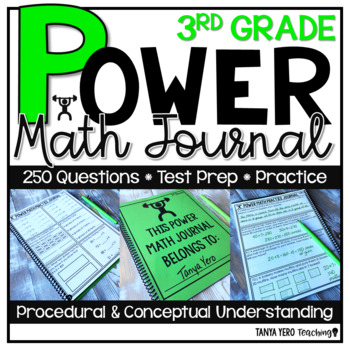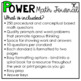# 3rd Grade Math Word Problems | Test Prep | Spiral Review | Morning WorkSubject
Resource Type
Common Core Standards
Product Rating
4.0
6 Ratings
File Type

PDF (Acrobat) Document File

3 MB|100 pages
Share
Also included in:
1. The POWER Math Ultimate Bundle is everything you need for a successful year of math instruction! The resources found in this bundle were designed with the philosophy in mind that math should be POWERful. POWER stands for purposeful opportunities with engagement and rigor. You and your students deser
\$108.42
\$78.00
Save \$30.42
Product Description

Add rigor and conceptual thinking to your math block with the POWER Math Journal. Designed for student practice, this journal has questions that target both procedural and conceptual understanding. You can bind the pages to make a journal or you can simply print out the pages for a print and go resource! 10 prompts/ questions are included per standard with space for work and answers. Most questions are short answer responses. You can use this math resource for test prep, math instruction, practice, or review! Answer keys are included.

What's included in this product?

• 250 procedural and conceptual based math questions
• Quality prompts and word problems that promote rigorous thinking
• Space for showing work and answers
• 10 questions per standard
• Each standard is formatted to two pages
• Easy formatting options - bind into a spiral book for a resource throughout the year or print pages as you go
• Easy prep

Perfect for your math lessons and in class practice.

WHAT ARE P.O.W.E.R PROBLEMS?

PURPOSEFUL - These problems are meant to keep students focused, while strengthening initiative and perseverance.

OPPORTUNITIES - These prompts can be used in a variety of ways. P.O.W.E.R problems can be used to introduce a lesson, spiral review, or as formative assessments.

WITH

ENGAGEMENT - Problems are real world applicable and designed to hook students with interest and presentation. Complexity of problems promotes problem solving skills.

RIGOR - Tasks are specifically designed to challenge students and assess conceptual understanding of curriculum versus procedural understanding. Students will need to apply more than just a “formula.”

WHY USE P.O.W.E.R PROBLEMS?

P.O.W.E.R problems are designed to challenge your students with their open ended presentation. Majority of problems that come from textbooks and workbooks assess procedural understanding of curriculum. Some textbooks even provide step by step instructions where the textbook is thinking for the students and taking away that “productive struggle” for children. When we rob students of that event, we rob them of their ability to reason, problem solve, and see beyond a standard algorithm. P.O.W.E.R problems are meant to show students that there are different ways to answer one question in math. With these tasks students take ownership and are part of the problem solving process versus filling in blanks in a textbook.

Standards & Topics Covered:

Number and Operation in Base Ten

➥ 3.NB.1 - Place value concepts

➥ 3.NBT.2 - Adding & subtracting whole numbers

➥ 3.NBT.3 – Multiplying numbers

Operations & Algebraic Thinking

➥ 3.OA.1 - Interpreting products of whole numbers

➥ 3.OA.2 – Interpreting quotients of whole numbers

➥ 3.OA.3 – Use multiplication and division to solve word problems

➥ 3.OA.4 – Determining unknown numbers in a multiplication or division equation

➥ 3.OA.5 – Apply properties of operations to multiply and divide

➥ 3.OA.6 – Understand division as an unknown-factor problem.

➥ 3.OA.7 – Fluently multiply and divide within 100

➥ 3.OA.8 – Solve two-step word problems using the four operations.

➥ 3.OA.9 – Understanding patterns on a multiplication chart

Number and Operation - Fractions

➥ 3.NF.1 – Understanding fractions

➥ 3.NF.2 – Understanding fractions on number lines

➥ 3.NF.3 – Equivalent fractions and comparting fractions

Measurement and Data

➥ 3.MD.1 – Understanding time

➥ 3.MD.2 – measuring and understanding liquid volume and mass

➥ 3.MD.3 – Picture graphs

➥ 3.MD.4 – Measuring length and using line plots

➥ 3.MD.5 – Understanding area

➥ 3.MD.6 - Measuring area

➥ 3.MD.7 - Relate area to the operations of multiplication and addition.

➥ 3.MD.8 – Word problems with area and perimeter

Geometry

➥ 3.G.1 – Understanding and examining shapes

➥ 3.G.2 - Partition shapes into parts with equal areas.

Total Pages
100 pages